# Measuring Length

Go back to  'Mensuration and Measurement'

## Measurement of length

The concept of measurement begins by making children understand the different parameters on which objects are measured. In Grade K children learn how an object can be described using its length, weight and capacity using simple terms like heavy & light, tall & short, big & small. An understanding of these concepts would set the stage for understanding the measurement of objects using different units.

-    Long and short - The image shows comparison of length.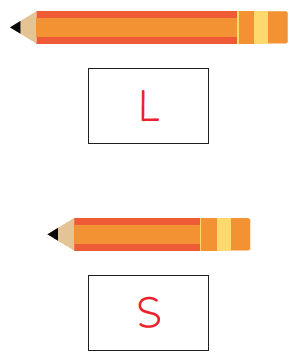-    Tall and short - The image shows comparison of height.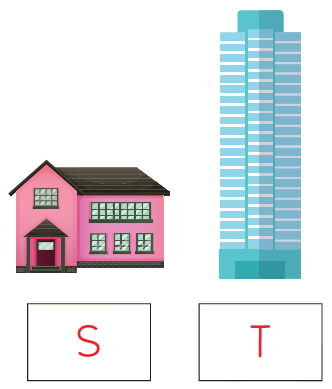-    Big and small - The image shows comparison of size.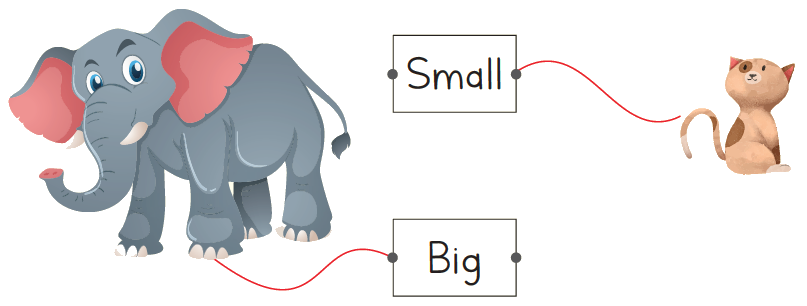## Non-standard units for measuring length

Non-standard units of measurement are used in early years of learning, to introduce children to the concept of measuring without them having to read any scales. Reading scales of any kind is a difficult skill in itself, so the idea of non-standard measures is to focus the child on the concept of heavier, lighter, longer, shorter, etc. before they move onto the next step of measuring using standard units.

To measure the length of the objects the non-standard measure used is that of hand spans. Using a handspan to measure objects is an informal way of figuring out the lengths. However, the measurements may vary owing to the subjectivity involved.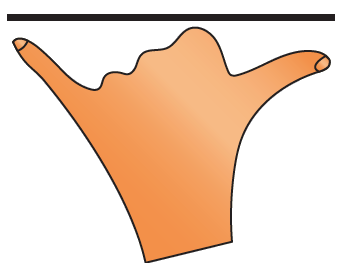## Standard units for measuring length

Children learn to use standard units of measurement in Grade 2, They learn about the tools and units appropriate for different objects. For example: The length of a pencil can be measured in centimetres using a ruler,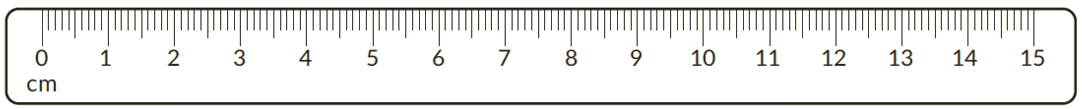Each number marks a ‘centimetre’ or ‘cm’. This ruler has 15 centimetres. The length of the given pencil is 8cm as shown on the ruler.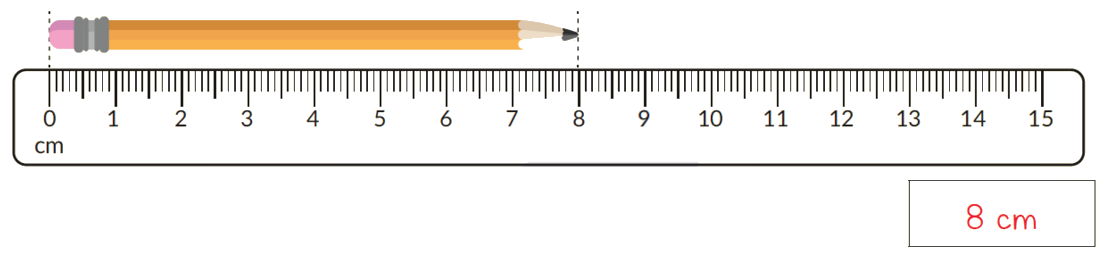### Formula

Children also learn about the relationship between units of measurement. They would need to know the following facts:

100 millimetres = 1 centimetre (cm)
100 centimetres = 1 metre (m)
1000 metres = 1 Kilometre km)

Using this fact, they would be able to convert the lengths. Add and subtract lengths. A thorough understanding of the conversion of units can be built by exposing children to real life situations where measuring converting and figuring out the solution happens.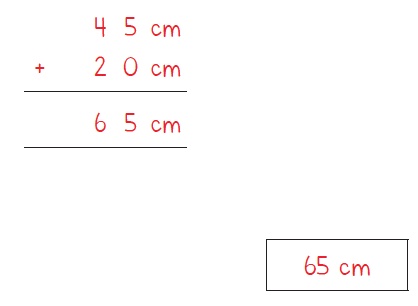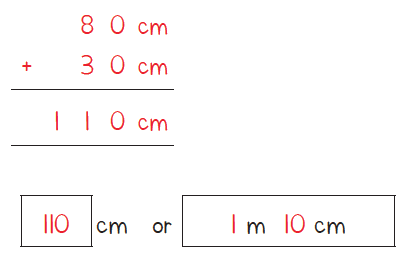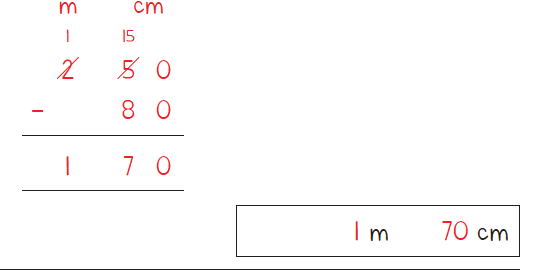Children would continue this kind of problem-solving in until Grade 5. They would solve application based problems involving all four operations (addition, subtraction, multiplication and division).

## Tips and Tricks

• Tip: Keep in mind that when converting from "cm" to "m" the conversion factor is only 100. From millimeter (mm) to meter (m) and from meter (m) to kilometre (km) the conversion factor is 1000.
• 1 km 25 m is often mistakenly written as 1.25 km instead of 1.025 km. Be careful with this.
• In most school curricula, the length conversions that students are expected to know are between mm, cm, m and km. For that use this diagram as the conversion progression is 10, then 100 and finally 1000.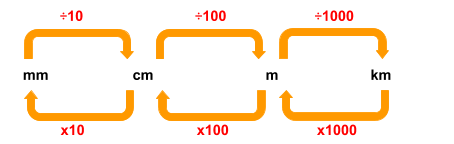Q1. Convert

a.  32 m = ________ cm
b.  6000 m = ______ km
c.  380 cm = ______ m  ____ cm

Q2. Compare. Write the correct sign (>, <, =)

a.  365 cm ____  2 m
b.  8 m 52 cm _____ 8052 cm
c.  5 km 380 m ____ 6 km

Measurement of Length
Measurement of Length
Measurement of length, weight, and capacity
Measurement of length, weight, and capacity
An Introduction to Measurement